##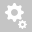Recently viewed tickets• Sales
• Library Model Request
• Technical Support

# How to obtain the sequence impedances from the Z matrix

To obtain the sequence impedance from the Z matrix, transpose the impedance matrix (Z) first:

Impedance Matrix: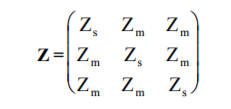Symmetrical Component Matrix: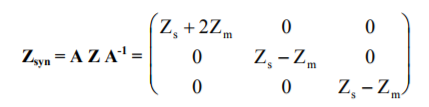Then the sequence impedances are as follows: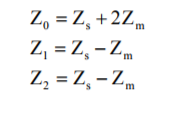Example:

Given the impedance matrix of a cable: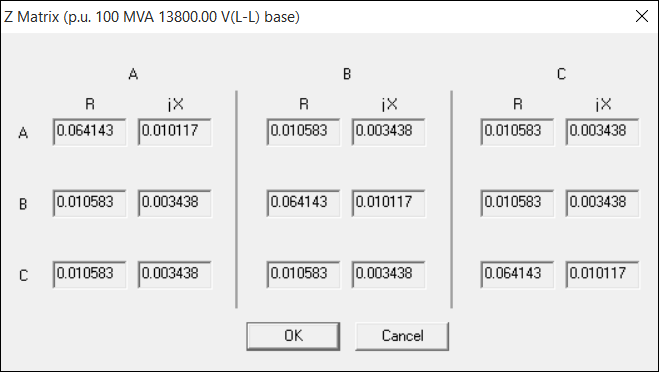Zs = 0.064143 + j0.010117
Zm = 0.010583 + j0.003438

Thus,
Z0 = (0.064143 + j0.010117) + 2*(0.010583 + j0.003438)
Z0 = 0.0853 + j0.017

Z0 = R0 + jX0

Z1 = (0.064143 + j0.010117) - (0.010583 + j0.003438)
Z1 = 0.0536 + j0.0067

Z1 = R1 + jX1

These are the same results shown on the cable datablock: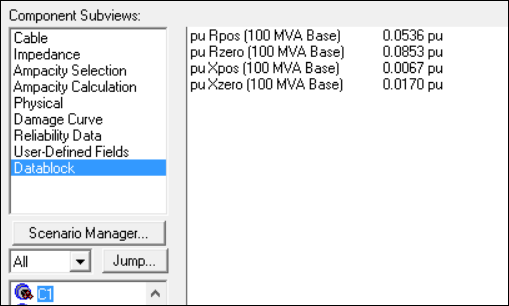Creation date: 8/15/2017 11:17 AM ()      Updated: 8/15/2017 11:17 AM ()